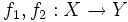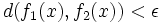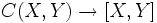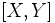# Close maps are homotopic

## Statement

Suppose$X$ is a topological space and$Y$ is a compact subset in Euclidean space, such that there exists an open subset$U \supset Y$ such that$Y$ is a strong deformation retract of$U$. Then, there exists$\epsilon > 0$ such that if two maps$f_1,f_2:X \to Y$ are$\epsilon$-close, in the sense:$d(f_1(x), f_2(x)) < \epsilon$

(where$d$ denotes the Euclidean distance) then$f_1$ and$f_2$ are homotopic.

## Alternative interpretations

A concrete interpretation of this is as follows. Suppose we view$Y$ as a compact metric space with the metric induced as a subset of$\R^n$. Then we can give$C(X,Y)$ the topology of uniform convergence. There is a natural map:$C(X,Y) \to [X,Y]$

where$[X,Y]$ denotes the space of homotopy classes of continuous maps from$X$ to$Y$. The above result says that the above map is continuous if we give$[X,Y]$ the discrete topology. This interpretation follows because for every function in a homotopy class, the$\epsilon$-neighbourhood of that function is also in the same homotopy class.

The advantage of this interpretation is that for$Y$ a compact metric space, the topology of uniform convergence coincides with the compact-open topology, which can be defined without reference to the explicit metric. Thus, we can state the result more abstractly as:

If$X$ is a topological space and$Y$ is a compact metrizable space, give$C(X,Y)$ the compact-open topology and$[X,Y]$ the discrete topology. Then the mapping:$C(X,Y) \to [X,Y]$

is continuous.

## Converse

A converse to this statement exists, but under different hypotheses; we need to assume that the space$X$ is compact and$Y$ just needs to be a metric space. Fill this in later